# Calculating Estimated Strip Widths - Roll Formed Cross-Sections

All roll formed cross-sections consist of at least one bend and most have a combination of bends and flats. The process of calculating a strip (blank) width essentially involves estimating the arc length (neutral line) of the bends and adding the sum of these bends to the lengths of all flat surfaces associated with the finished cross-section.

In roll forming, a bend is formed progressively until the final forming angle has been achieved. Bending metal places the inside bend radius under a compressive state while at the same time the outer bend radius elongates.  Somewhere between the inner and outer bend radius is a portion of the material that remains unaffected (or neutral) by forming. This is the true arc length of the bend that needs to be predicted.  Although in theory the outside radius will be exactly one material thickness greater than the inside radius of a bend, there is normally a slight thinning (necking) which occurs naturally. This can lead to problems in calculating the correct strip width (Figure 1). When thinning occurs, excessive material that is not consumed within the bend angle is usually displaced into the adjacent flat, and may result in a burr or other defect. This thinning is generally more pronounced with material that is 0.060” and thicker, or when the inside bend radius is smaller than the recommended ASTM specification for the given material type and grade. Thinning can also be caused by the material properties, complexity of the cross-sections itself or the way the rolls are designed. In these cases, additional considerations must be made to minimize the potential errors in the calculation and final part geometry.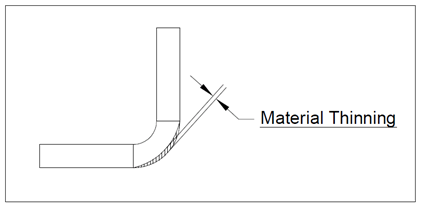Figure 1:  Material thinning around the outer surface of the radius

The following example illustrates the proper calculation of a strip width.

Determining the neutral line to calculate the total arc length of a bend:

Most metals have mechanical properties that a roll designer considers when designing the tooling. The three critical properties are yield strength, ultimate tensile strength and elongation (%). When calculating a strip width, the general rule of thumb is to use between 35-40% of material thickness to determine the bend allowance for material that is between 30,000-50,000 PSI yield strength. For materials that have yield strengths higher than 50,000 PSI, the designer may use a bend allowance between 45-50% of material thickness. In Figure 2, the angle profile has one bend and two flat surfaces on either side. The inside radius is 0.250”, the bend angle is 90 degrees and the material thickness is 0.250”.  Figure 3 shows the calculation of bend allowance and arc length.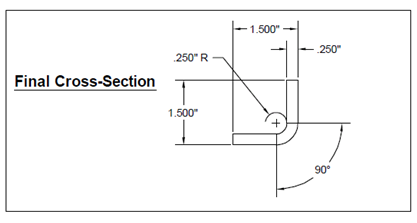Figure 2: Final cross-section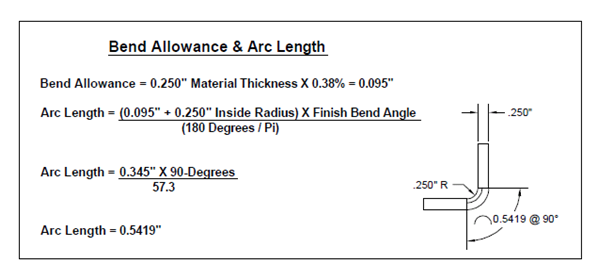Figure 3:  Calculating the bend allowance & arc length

Now that the arc length has been calculated, the remaining flat surfaces of the profile can be added to this for an estimated strip width. In Figure 4, the flat lengths are derived by subtracting the material thickness and inside bend radius from the 1.500” overall part height and width.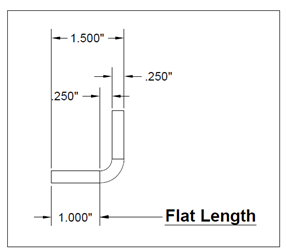Figure 4:  Calculating the flat surfaces of the profile
In Figure 5, the sum of the two flat surface lengths and the arc length of the bend equals the estimated strip width of the angle profile.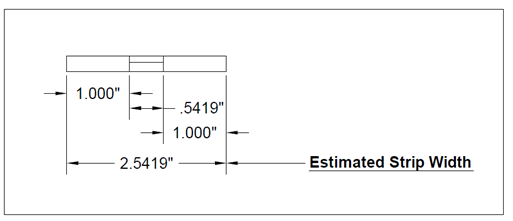Figure 5:  Estimated strip width
There are many other examples of roll formed cross sections that require additional explanation. These will be issued in later editions of the Roll-Kraft Roll Forming Pharmacy e-newsletter.

Author: Chuck Summerhill

Use our contact form below to request more information on tube and pipe or roll forming products. All of our products and services have a 100% performance guarantee. If you are not satisfied in any way you will receive a free replacement or a complete refund.